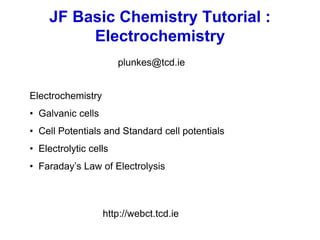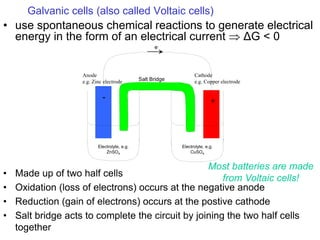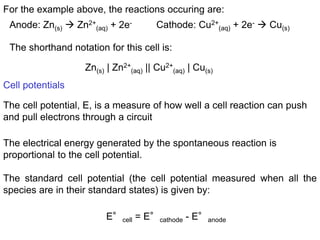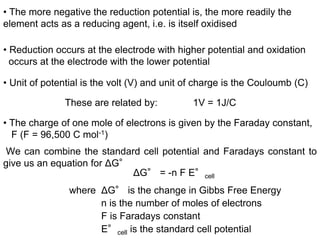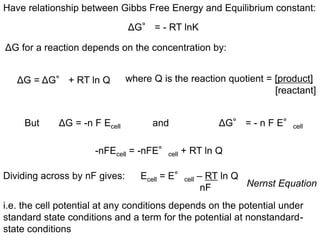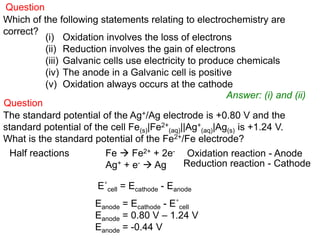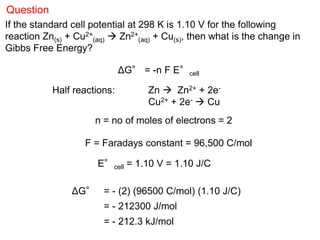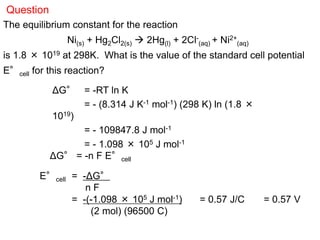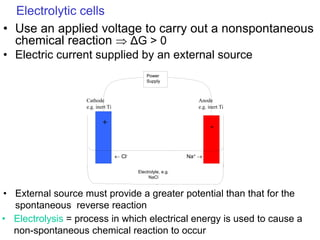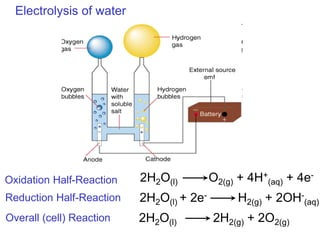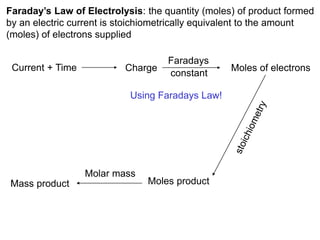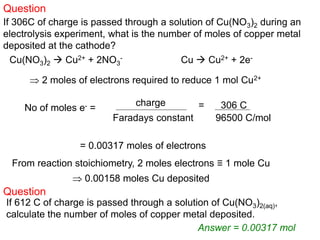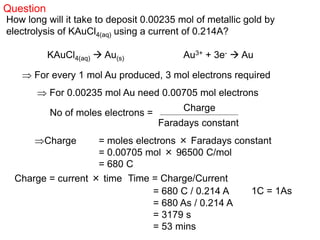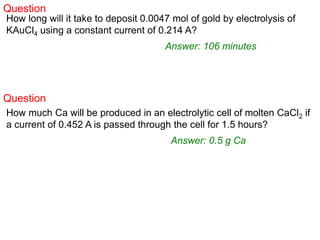1 of 14

### Tutorial 5 - Electrochemistry.ppt

1. JF Basic Chemistry Tutorial : Electrochemistry Electrochemistry • Galvanic cells • Cell Potentials and Standard cell potentials • Electrolytic cells • Faraday’s Law of Electrolysis http://webct.tcd.ie plunkes@tcd.ie
2. e- Cathode e.g. Copper electrode Anode e.g. Zinc electrode Salt Bridge Electrolyte, e.g. ZnSO4 Electrolyte, e.g. CuSO4 - + Galvanic cells (also called Voltaic cells) • use spontaneous chemical reactions to generate electrical energy in the form of an electrical current  ΔG < 0 • Made up of two half cells • Oxidation (loss of electrons) occurs at the negative anode • Reduction (gain of electrons) occurs at the postive cathode • Salt bridge acts to complete the circuit by joining the two half cells together Most batteries are made from Voltaic cells!
3. For the example above, the reactions occuring are: Anode: Zn(s)  Zn2+ (aq) + 2e- Cell potentials The electrical energy generated by the spontaneous reaction is proportional to the cell potential. The standard cell potential (the cell potential measured when all the species are in their standard states) is given by: E°cell = E°cathode - E°anode Cathode: Cu2+ (aq) + 2e-  Cu(s) The shorthand notation for this cell is: Zn(s) | Zn2+ (aq) || Cu2+ (aq) | Cu(s) The cell potential, E, is a measure of how well a cell reaction can push and pull electrons through a circuit
4. • Reduction occurs at the electrode with higher potential and oxidation occurs at the electrode with the lower potential • Unit of potential is the volt (V) and unit of charge is the Couloumb (C) These are related by: 1V = 1J/C • The charge of one mole of electrons is given by the Faraday constant, F (F = 96,500 C mol-1) • The more negative the reduction potential is, the more readily the element acts as a reducing agent, i.e. is itself oxidised We can combine the standard cell potential and Faradays constant to give us an equation for ΔG° ΔG° = -n F E°cell where ΔG° is the change in Gibbs Free Energy n is the number of moles of electrons F is Faradays constant E°cell is the standard cell potential
5. Have relationship between Gibbs Free Energy and Equilibrium constant: ΔG° = - RT lnK ΔG for a reaction depends on the concentration by: ΔG = ΔG° + RT ln Q where Q is the reaction quotient = [product] [reactant] But ΔG = -n F Ecell and ΔG° = - n F E°cell Dividing across by nF gives: Ecell = E°cell – RT ln Q nF Nernst Equation -nFEcell = -nFE°cell + RT ln Q i.e. the cell potential at any conditions depends on the potential under standard state conditions and a term for the potential at nonstandard- state conditions
6. Question Which of the following statements relating to electrochemistry are correct? (i) Oxidation involves the loss of electrons (ii) Reduction involves the gain of electrons (iii) Galvanic cells use electricity to produce chemicals (iv) The anode in a Galvanic cell is positive (v) Oxidation always occurs at the cathode Answer: (i) and (ii) The standard potential of the Ag+/Ag electrode is +0.80 V and the standard potential of the cell Fe(s)|Fe2+ (aq)||Ag+ (aq)|Ag(s) is +1.24 V. What is the standard potential of the Fe2+/Fe electrode? Question Half reactions Fe  Fe2+ + 2e- Ag+ + e-  Ag Oxidation reaction - Anode Reduction reaction - Cathode E˚cell = Ecathode - Eanode Eanode = Ecathode - E˚cell Eanode = 0.80 V – 1.24 V Eanode = -0.44 V
7. Question If the standard cell potential at 298 K is 1.10 V for the following reaction Zn(s) + Cu2+ (aq)  Zn2+ (aq) + Cu(s), then what is the change in Gibbs Free Energy? ΔG° = -n F E°cell n = no of moles of electrons = 2 F = Faradays constant = 96,500 C/mol E°cell = 1.10 V = 1.10 J/C ΔG° = - (2) (96500 C/mol) (1.10 J/C) = - 212300 J/mol = - 212.3 kJ/mol Half reactions: Zn  Zn2+ + 2e- Cu2+ + 2e-  Cu
8. The equilibrium constant for the reaction Ni(s) + Hg2Cl2(s)  2Hg(l) + 2Cl- (aq) + Ni2+ (aq) is 1.8 × 1019 at 298K. What is the value of the standard cell potential E°cell for this reaction? ΔG° = -RT ln K = - (8.314 J K-1 mol-1) (298 K) ln (1.8 × 1019) = - 109847.8 J mol-1 = - 1.098 × 105 J mol-1 ΔG° = -n F E°cell E°cell = -ΔG° n F = -(-1.098 × 105 J mol-1) = 0.57 J/C = 0.57 V (2 mol) (96500 C) Question
9. Na+   Cl- Anode e.g. inert Ti Cathode e.g. inert Ti Electrolyte, e.g. NaCl + - Power Supply Electrolytic cells • Use an applied voltage to carry out a nonspontaneous chemical reaction  ΔG > 0 • Electric current supplied by an external source • External source must provide a greater potential than that for the spontaneous reverse reaction • Electrolysis = process in which electrical energy is used to cause a non-spontaneous chemical reaction to occur
10. Electrolysis of water 2H2O(l) O2(g) + 4H+ (aq) + 4e- Oxidation Half-Reaction Overall (cell) Reaction Reduction Half-Reaction 2H2O(l) + 2e- H2(g) + 2OH- (aq) 2H2O(l) 2H2(g) + 2O2(g)
11. Current + Time Charge Faradays constant Moles of electrons Moles product Molar mass Mass product Using Faradays Law! Faraday’s Law of Electrolysis: the quantity (moles) of product formed by an electric current is stoichiometrically equivalent to the amount (moles) of electrons supplied
12. Question If 306C of charge is passed through a solution of Cu(NO3)2 during an electrolysis experiment, what is the number of moles of copper metal deposited at the cathode? Cu(NO3)2  Cu2+ + 2NO3 - Cu  Cu2+ + 2e-  2 moles of electrons required to reduce 1 mol Cu2+ No of moles e- = charge Faradays constant = 306 C 96500 C/mol = 0.00317 moles of electrons From reaction stoichiometry, 2 moles electrons ≡ 1 mole Cu  0.00158 moles Cu deposited Question If 612 C of charge is passed through a solution of Cu(NO3)2(aq), calculate the number of moles of copper metal deposited. Answer = 0.00317 mol
13. How long will it take to deposit 0.00235 mol of metallic gold by electrolysis of KAuCl4(aq) using a current of 0.214A? Charge = current × time KAuCl4(aq)  Au(s) Au3+ + 3e-  Au  For every 1 mol Au produced, 3 mol electrons required  For 0.00235 mol Au need 0.00705 mol electrons No of moles electrons = Faradays constant Charge Question Charge = moles electrons × Faradays constant = 0.00705 mol × 96500 C/mol = 680 C Time = Charge/Current = 680 C / 0.214 A 1C = 1As = 680 As / 0.214 A = 3179 s = 53 mins
14. Question How long will it take to deposit 0.0047 mol of gold by electrolysis of KAuCl4 using a constant current of 0.214 A? Answer: 106 minutes Question How much Ca will be produced in an electrolytic cell of molten CaCl2 if a current of 0.452 A is passed through the cell for 1.5 hours? Answer: 0.5 g Ca